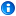### Scope of block variablesThis problem is fixed in Ruby 1.9. Block parameters are strictly local.

Consider the following code.

```x = 0
a = [1, 2, 3]
a.each { |x| }
puts 'x=' + x.to_s
```

One should naturally expect that x in the block following "a.each" is local to that block and that the output of this code will be "x=0". Yet the actual output is "x=3" as of ruby 1.8.7.

So at this point one should again naturally conclude that x is global so that the following code will print "x=3".

```a = [1, 2, 3]
a.each { |x| }
puts 'x=' + x.to_s
```

But the actual output is

my.rb:3: undefined local variable or method `x' for main:Object (NameError)

The confusing matter of fact is, for a block variable x,

1. if x is already defined, it refers to that variable;
2. if x is not yet defined, a new variable that is local to the block is created.

### Precedence of or, and, &&, ||

Consider the following irb session:

irb(main):001:0> true || true && false
=> true
irb(main):002:0> true or true and false
=> false
irb(main):003:0> false and true || true
=> false

Only the first line meets one's intuition, because "&&" has higher precedence than "||".

The 2nd and 3rd happens because "and" and "or" has the same precedence, and "||" has higher precedence than "and".Document generated by Confluence on Jan 27, 2014 16:56